#### You may also like### Real(ly) Numbers

If x, y and z are real numbers such that: x + y + z = 5 and xy + yz + zx = 3. What is the largest value that any of the numbers can have?In y = ax +b when are a, -b/a, b in arithmetic progression. The polynomial y = ax^2 + bx + c has roots r1 and r2. Can a, r1, b, r2 and c be in arithmetic progression?### Two Cubes

Two cubes, each with integral side lengths, have a combined volume equal to the total of the lengths of their edges. How big are the cubes? [If you find a result by 'trial and error' you'll need to prove you have found all possible solutions.]

# Curve Fitter

##### Age 14 to 18Challenge Level

Part 1
Sammy from Lancing College in the UK used the general form of a cubic equation to find some examples: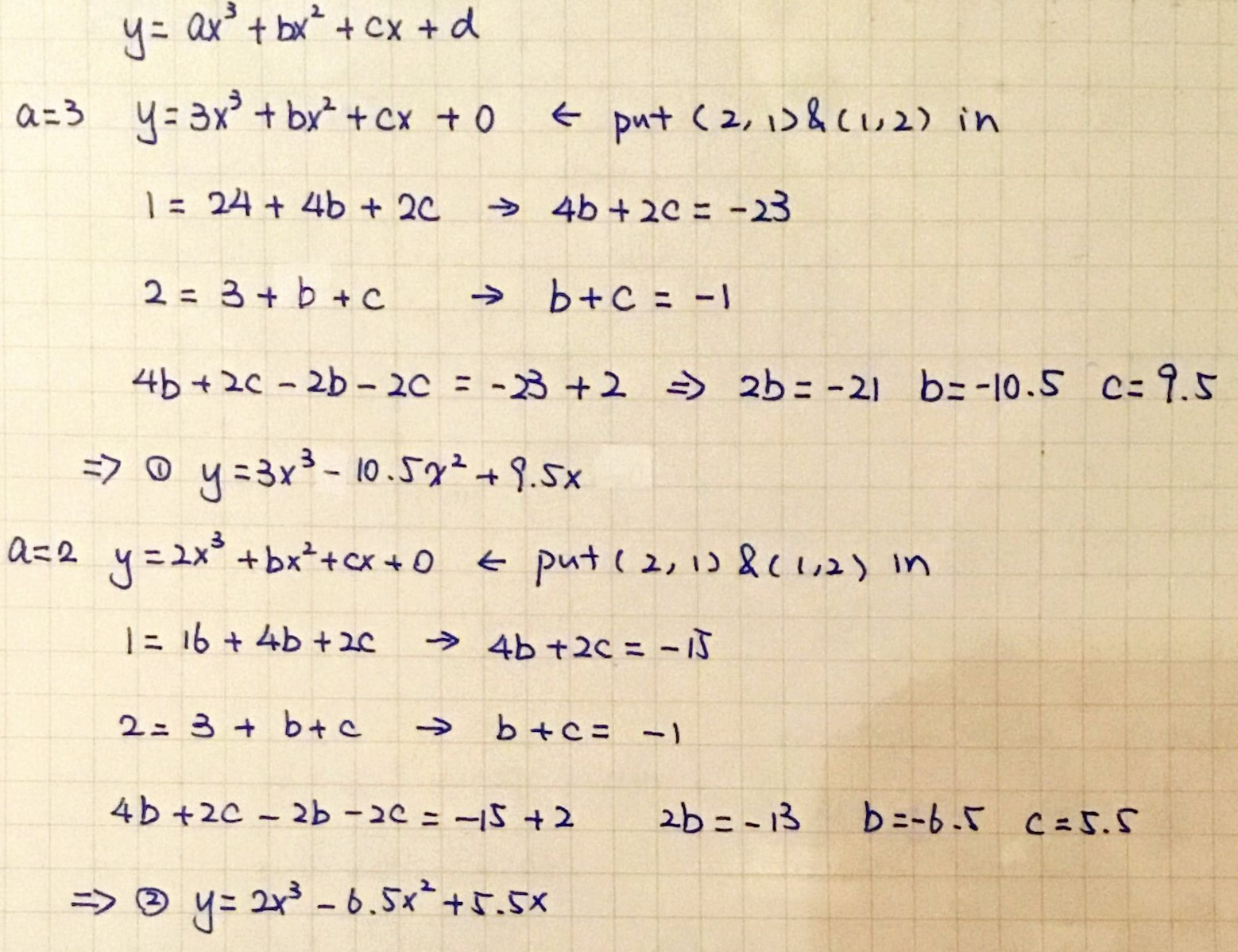Mahdi from Mahatma Gandhi International School in India found the general form of a cubic which passes through the three points: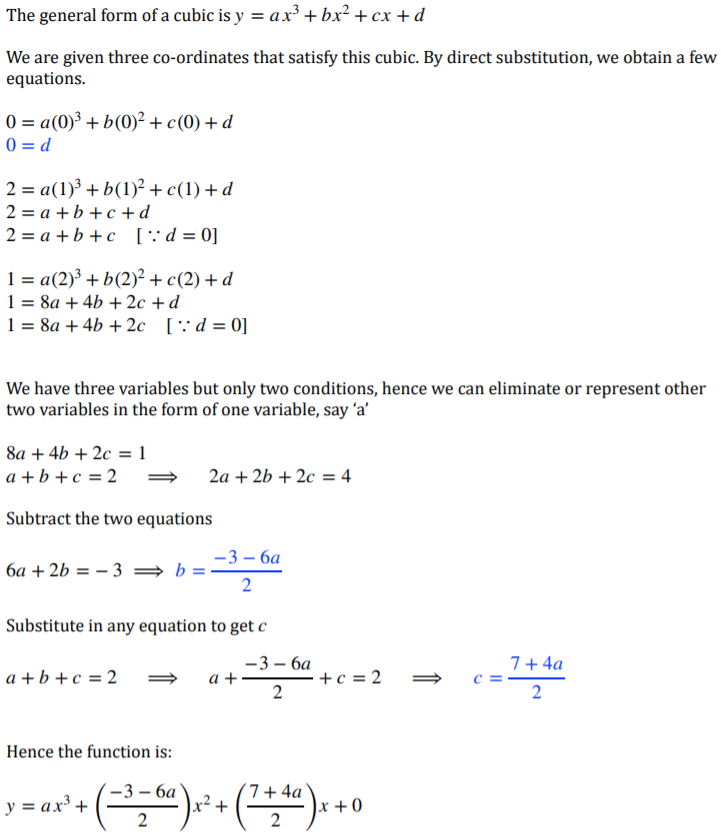Mahdi also created a video showing all of the possible cubics. Click here to download Mahdi's video. Notice how similar this is to what happens on the GeoGebra page in the link in the hint of the problem.

Part 2
Siddhant from Singapore International School in India and Sammy used the same setup Sammy used in part 1 to find a cubic that works for part 2a. This is Siddhant's work:
The general form of a cubic curve is $$y = ax^3 + bx^2 + cx + d$$
$(0,0) (1,2) (2,1)$
To find the values of $a, b, c$ and $d,$ we just need to substitute the values of $x$ and $y$ in each of the three coordinates, and build a system of three equations. These equations are:
$(0,0)$
$0 = d$

$(1,2)$
$2 = a + b + c$

$(2,1)$
$1 = 8a + 4b + 2c$

We also know that $(1,2)$ is a stationary point. This tells us that the first derivative of the general equation is equal to $0$ at $x=1.$
first derivative: $3ax^2 + 2bx + c$
Therefore: $3a(1)^2 + 2b(1) + c = 0$
$3a + 2b + c = 0$

Thus, the three equations we have are,
1. $a + b + c = 2$
2. $8a + 4b + 2c = 1$
3. $3a + 2b + c= 0$

We can multiply equation 3 by $2,$ and then subtract that from equation 2.
This gives us,
$2a = 1$
$a = \frac12$

From this, we get the two equations:
$b + c = \frac32$
$4b + 2c = -3$

By using elimination method, the values of $b$ and $c$ are,
$b = -3\\ c = 4.5$

Therefore, the cubic equation we get is:
$y = \frac12x^3 -3x^2 + 4.5x$

Mahdi used the general cubic from part 1 and also combined this with differentiation:
Equation: $y = ax^3 + \dfrac{-3-6a}{2}x^2 + \dfrac{7+4a}{2}x$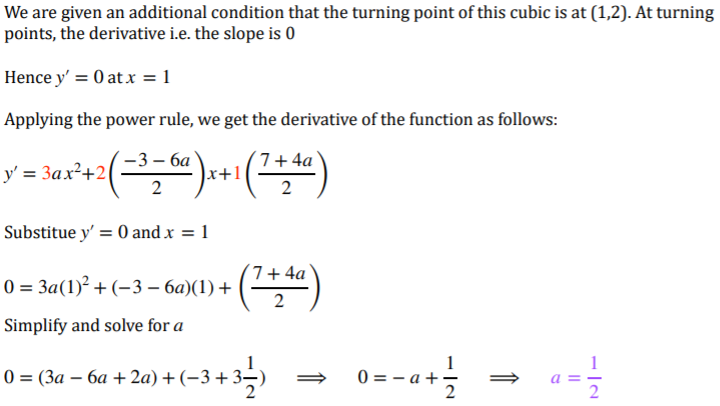Which also gives the equation $y = \frac12 x^3 -3x^2 + \frac92 x$

Mahdi also found a curve for part 2b: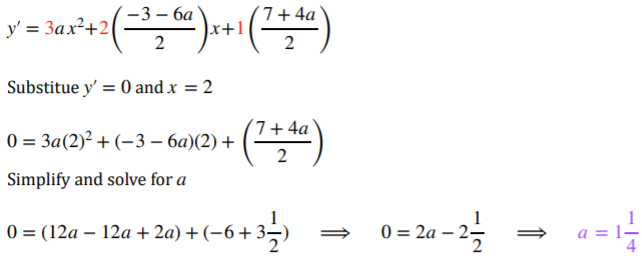Which gives the equation $y = 1\frac14 x^3 -5\frac14 x^2 + 6x$

Part 3
Mahdi explained why this is not possible using the results from part 2: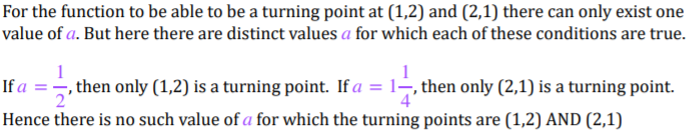This is Sammy's work for part 3. Sammy began with the general equation for a cubic which passes through $(0,0)$ and then chose the turning points to be when $x=1$ and when $x=2.$ However, Sammy's conclusion is not correct (see explanation below):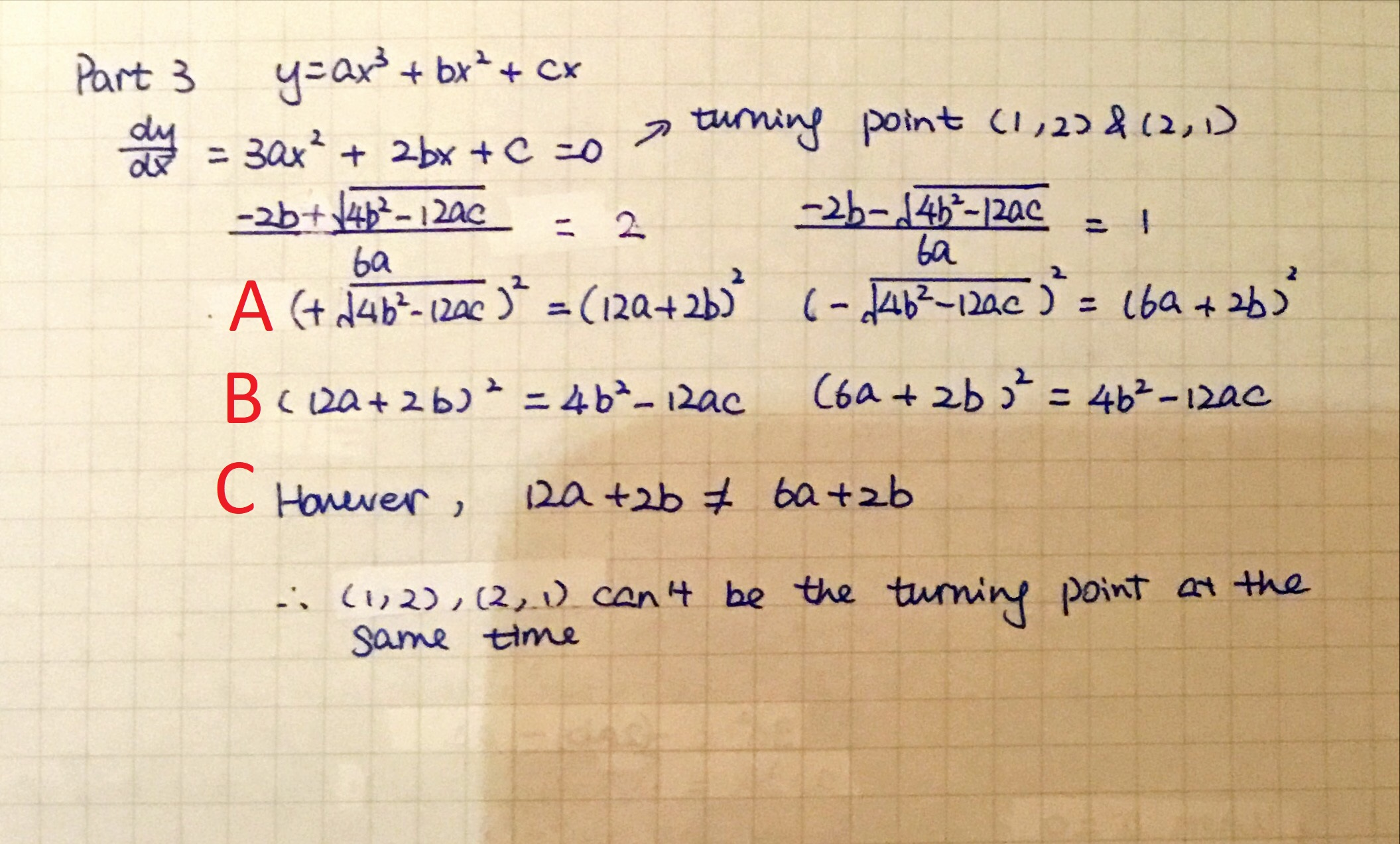Between lines A and B, Sammy loses information, because $12a+2b$ was supposed to be the positive square root whereas $6a+2b$ was the negative square root. This information is lost when both sides are squared.

In line C, having lost the information about the negative square root, Sammy gets that $12a+2b=6a+2b$ and notices that this would give $a=0,$ which isn't allowed. However, using the negative square root information, this should be $12a+2b=-(6a+2b)\Rightarrow18a=-4b\Rightarrow b=\frac{-9}{2}a.$

You can substitute this back into Sammy's earlier equations to find $c$ in terms of $a$ (or vice versa) and therefore find a family of cubics which go through $(0,0)$ and have turning points at $x=1$ and $x=2.$ However, as Siddhant and Mahdi have shown, it won't include one with $y=2$ when $x=1$ and $y=1$ when $x=2.$ Can you prove it using this method?